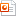검색어 입력폼

# [MATLAB] Euler Method

저작시기 2009.06 |등록일 2009.06.17MS 파워포인트 (ppt) | 14페이지 | 가격 2,000원

오일러 방법

## 목차

Euler’s Method 란 무엇인가?
Problem 2.
Analytic 한 풀이
matlab code.
compare
Conclusion

## 본문내용

*Euler Method
y’ = f(x,y) , y(x0)=y0

L(x) = y0 + f(x0,y0)(x-x0)

We now let ‘h’ be a positive increment of the x- axis, as shown in this figure.

Then by replacing x by x1=x0+h, we get
L(x1) = y0 + f(x0,y0)(x0+h-x0) or
y1 = y0 + hf(x1,y1) , where y1 = L(x1)
the point tangent line is an approximation to the point (x1,y(x1)) on the solution curve.

Continuing in this manner,
yn+1 = yn + hf(xn, yn) where xn=x0+nh (n=0,1,2,…..)

This procedure of using successive “tangent lines” is called Euler’s Method.

없음